• 前一篇文章讲解了图像灰度化处理及线性变换知识，结合OpenCV调用cv2.cvtColor()函数实现图像灰度操作，本篇文章主要讲解非线性变换，使用自定义方法对图像进行灰度化处理，包括对数变换和伽马变换。本文主要讲解灰度...
该系列文章是讲解Python OpenCV图像处理知识，前期主要讲解图像入门、OpenCV基础用法，中期讲解图像处理的各种算法，包括图像锐化算子、图像增强技术、图像分割等，后期结合深度学习研究图像识别、图像分类应用。希望文章对您有所帮助，如果有不足之处，还请海涵~
该系列在github所有源代码：https://github.com/eastmountyxz/ImageProcessing-Python
PS：请求帮忙点个Star，哈哈，第一次使用Github，以后会分享更多代码，一起加油。
同时推荐作者的C++图像系列知识：
[数字图像处理] 一.MFC详解显示BMP格式图片
[数字图像处理] 二.MFC单文档分割窗口显示图片
[数字图像处理] 三.MFC实现图像灰度、采样和量化功能详解
[数字图像处理] 四.MFC对话框绘制灰度直方图
[数字图像处理] 五.MFC图像点运算之灰度线性变化、灰度非线性变化、阈值化和均衡化处理详解
[数字图像处理] 六.MFC空间几何变换之图像平移、镜像、旋转、缩放详解
[数字图像处理] 七.MFC图像增强之图像普通平滑、高斯平滑、Laplacian、Sobel、Prewitt锐化详解
前文参考：
[Python图像处理] 一.图像处理基础知识及OpenCV入门函数
[Python图像处理] 二.OpenCV+Numpy库读取与修改像素
[Python图像处理] 三.获取图像属性、兴趣ROI区域及通道处理
[Python图像处理] 四.图像平滑之均值滤波、方框滤波、高斯滤波及中值滤波
[Python图像处理] 五.图像融合、加法运算及图像类型转换
[Python图像处理] 六.图像缩放、图像旋转、图像翻转与图像平移
[Python图像处理] 七.图像阈值化处理及算法对比
[Python图像处理] 八.图像腐蚀与图像膨胀
[Python图像处理] 九.形态学之图像开运算、闭运算、梯度运算
[Python图像处理] 十.形态学之图像顶帽运算和黑帽运算
[Python图像处理] 十一.灰度直方图概念及OpenCV绘制直方图
[Python图像处理] 十二.图像几何变换之图像仿射变换、图像透视变换和图像校正
[Python图像处理] 十三.基于灰度三维图的图像顶帽运算和黑帽运算
[Python图像处理] 十四.基于OpenCV和像素处理的图像灰度化处理
[Python图像处理] 十五.图像的灰度线性变换
前一篇文章讲解了图像灰度化处理及线性变换知识，结合OpenCV调用cv2.cvtColor()函数实现图像灰度操作，本篇文章主要讲解非线性变换，使用自定义方法对图像进行灰度化处理，包括对数变换和伽马变换。本文主要讲解灰度线性变换，基础性知识希望对您有所帮助。
1.图像灰度非线性变换：DB=DA×DA/255
2.图像灰度对数变换
3.图像灰度伽玛变换
PS：文章参考自己以前系列图像处理文章及OpenCV库函数，同时参考如下文献：
杨秀璋等. 基于苗族服饰的图像锐化和边缘提取技术研究[J]. 现代计算机，2018(10).
《数字图像处理》（第3版），冈萨雷斯著，阮秋琦译，电子工业出版社，2013年.
《数字图像处理学》（第3版），阮秋琦，电子工业出版社，2008年，北京.
《OpenCV3编程入门》，毛星云，冷雪飞，电子工业出版社，2015.
[数字图像处理] 五.MFC图像点运算之灰度线性变化、灰度非线性变化、阈值化和均衡化处理详解
python+opencv+图像特效（图像灰度处理、颜色翻转、图片融合，边缘检测，浮雕效果）
数字图像处理-空间域处理-灰度变换-基本灰度变换函数
OpenCV图像增强算法实现（直方图均衡化、拉普拉斯、Log、Gamma）

一.图像灰度非线性变换：DB=DA×DA/255
图像的灰度非线性变换主要包括对数变换、幂次变换、指数变换、分段函数变换，通过非线性关系对图像进行灰度处理，下面主要讲解三种常见类型的灰度非线性变换。
原始图像的灰度值按照DB=DA×DA/255的公式进行非线性变换，其代码如下：
# -*- coding: utf-8 -*-
import cv2
import numpy as np
import matplotlib.pyplot as plt

#读取原始图像

#图像灰度转换
grayImage = cv2.cvtColor(img, cv2.COLOR_BGR2GRAY)

#获取图像高度和宽度
height = grayImage.shape
width = grayImage.shape

#创建一幅图像
result = np.zeros((height, width), np.uint8)

#图像灰度非线性变换：DB=DA×DA/255
for i in range(height):
for j in range(width):
gray = int(grayImage[i,j])*int(grayImage[i,j]) / 255
result[i,j] = np.uint8(gray)

#显示图像
cv2.imshow("Gray Image", grayImage)
cv2.imshow("Result", result)

#等待显示
cv2.waitKey(0)
cv2.destroyAllWindows()

图像灰度非线性变换的输出结果下图所示：二.图像灰度对数变换
图像灰度的对数变换一般表示如公式所示：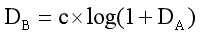其中c为尺度比较常数，DA为原始图像灰度值，DB为变换后的目标灰度值。如下图所示，它表示对数曲线下的灰度值变化情况。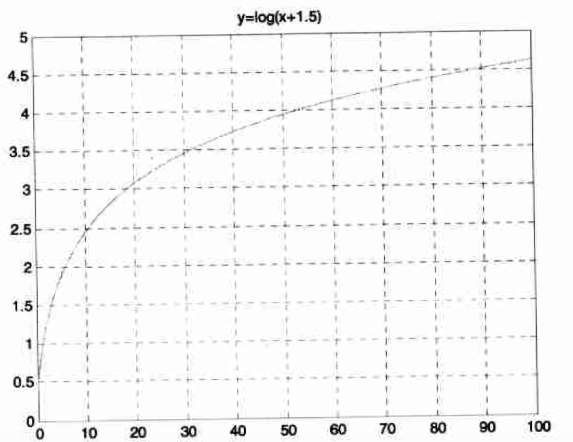由于对数曲线在像素值较低的区域斜率大，在像素值较高的区域斜率较小，所以图像经过对数变换后，较暗区域的对比度将有所提升。这种变换可用于增强图像的暗部细节，从而用来扩展被压缩的高值图像中的较暗像素。
对数变换实现了扩展低灰度值而压缩高灰度值的效果，被广泛地应用于频谱图像的显示中。一个典型的应用是傅立叶频谱，其动态范围可能宽达0～106直接显示频谱时，图像显示设备的动态范围往往不能满足要求，从而丢失大量的暗部细节；而在使用对数变换之后，图像的动态范围被合理地非线性压缩，从而可以清晰地显示。在下图中，未经变换的频谱经过对数变换后，增加了低灰度区域的对比度，从而增强暗部的细节。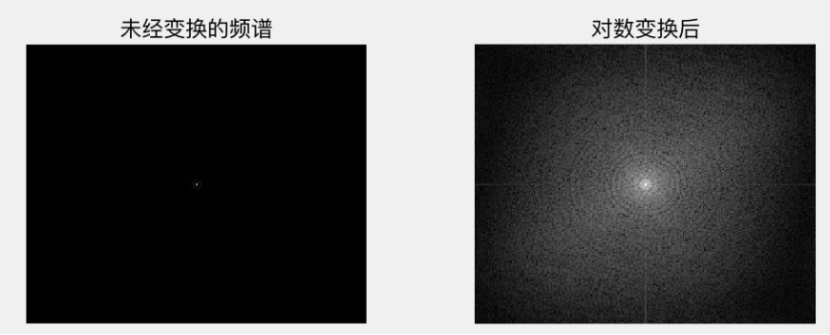下面的代码实现了图像灰度的对数变换。
# -*- coding: utf-8 -*-
import numpy as np
import matplotlib.pyplot as plt
import cv2

#绘制曲线
def log_plot(c):
x = np.arange(0, 256, 0.01)
y = c * np.log(1 + x)
plt.plot(x, y, 'r', linewidth=1)
plt.rcParams['font.sans-serif']=['SimHei'] #正常显示中文标签
plt.title(u'对数变换函数')
plt.xlim(0, 255), plt.ylim(0, 255)
plt.show()

#对数变换
def log(c, img):
output = c * np.log(1.0 + img)
output = np.uint8(output + 0.5)
return output

#读取原始图像

#绘制对数变换曲线
log_plot(42)

#图像灰度对数变换
output = log(42, img)

#显示图像
cv2.imshow('Input', img)
cv2.imshow('Output', output)
cv2.waitKey(0)
cv2.destroyAllWindows()

下图表示经过对数函数处理后的效果图，对数变换对于整体对比度偏低并且灰度值偏低的图像增强效果较好。对应的对数函数曲线如图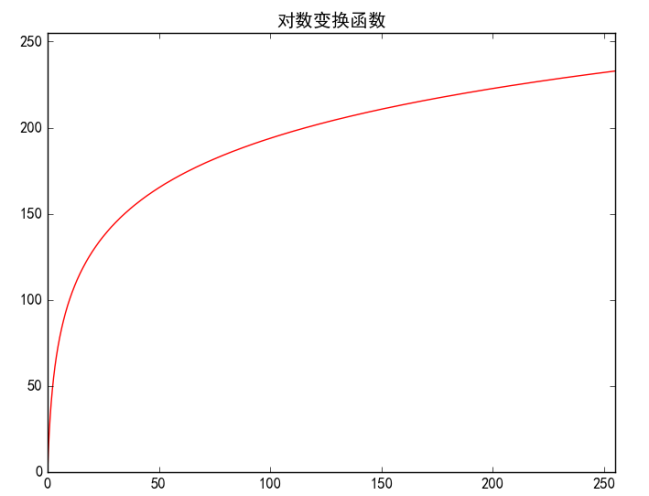三.图像灰度伽玛变换
伽玛变换又称为指数变换或幂次变换，是另一种常用的灰度非线性变换。图像灰度的伽玛变换一般表示如公式所示：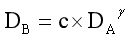当γ>1时，会拉伸图像中灰度级较高的区域，压缩灰度级较低的部分。
当γ<1时，会拉伸图像中灰度级较低的区域，压缩灰度级较高的部分。
当γ=1时，该灰度变换是线性的，此时通过线性方式改变原图像。

Python实现图像灰度的伽玛变换代码如下，主要调用幂函数实现。
# -*- coding: utf-8 -*-
import numpy as np
import matplotlib.pyplot as plt
import cv2

#绘制曲线
def gamma_plot(c, v):
x = np.arange(0, 256, 0.01)
y = c*x**v
plt.plot(x, y, 'r', linewidth=1)
plt.rcParams['font.sans-serif']=['SimHei'] #正常显示中文标签
plt.title(u'伽马变换函数')
plt.xlim([0, 255]), plt.ylim([0, 255])
plt.show()

#伽玛变换
def gamma(img, c, v):
lut = np.zeros(256, dtype=np.float32)
for i in range(256):
lut[i] = c * i ** v
output_img = cv2.LUT(img, lut) #像素灰度值的映射
output_img = np.uint8(output_img+0.5)
return output_img

#读取原始图像

#绘制伽玛变换曲线
gamma_plot(0.00000005, 4.0)

#图像灰度伽玛变换
output = gamma(img, 0.00000005, 4.0)

#显示图像
cv2.imshow('Imput', img)
cv2.imshow('Output', output)
cv2.waitKey(0)
cv2.destroyAllWindows()

下图表示经过伽玛变换处理后的效果图，伽马变换对于图像对比度偏低，并且整体亮度值偏高（或由于相机过曝）情况下的图像增强效果明显。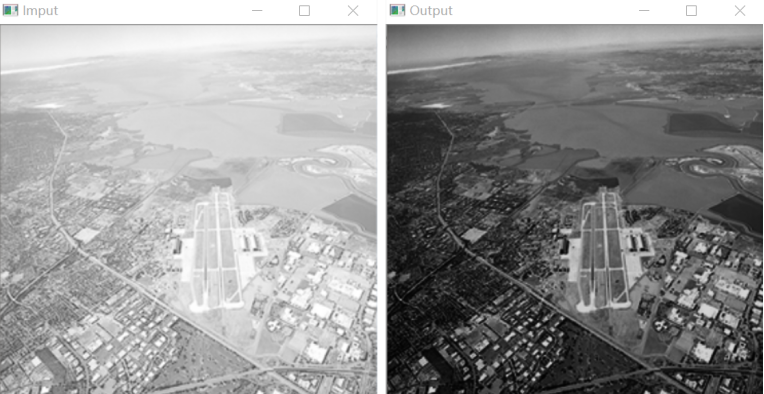对应的幂律函数曲线如图所示。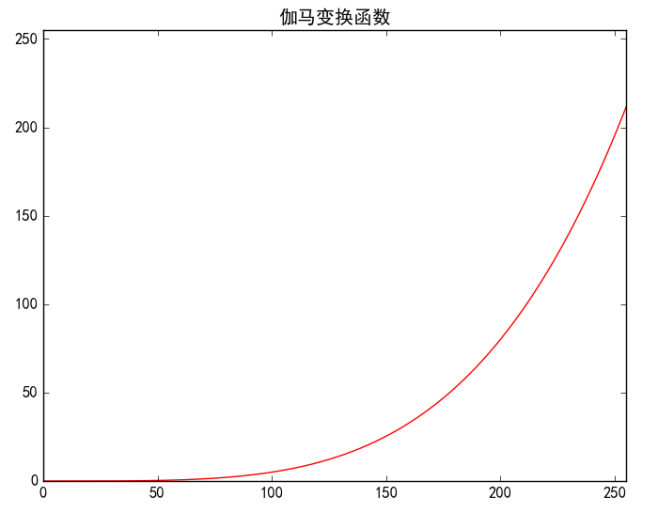文章周日写于钟书阁，女神伴于旁。希望文章对大家有所帮助，如果有错误或不足之处，还请海涵。最近连续奔波考博，经历的事情太多，有喜有悲，需要改变自己好好对女神，也希望读者与我一起加油。
（By：Eastmount 2019-03-31 深夜12点 https://blog.csdn.net/Eastmount/）

2020年8月18新开的“娜璋AI安全之家”，主要围绕Python大数据分析、网络空间安全、人工智能、Web渗透及攻防技术进行讲解，同时分享CCF、SCI、南核北核论文的算法实现。娜璋之家会更加系统，并重构作者的所有文章，从零讲解Python和安全，写了近十年文章，真心想把自己所学所感所做分享出来，还请各位多多指教，真诚邀请您的关注！谢谢。展开全文灰度非线性变换
• 图像处理中图像的点运算，包括图像配准，图像线性变换，对数变换，阈值变换等
• 设置窗口大小的函数： namedWindow("窗口名", 0); resizeWindow("窗口名", ... resizeWindow("对数变换", 500, 600); imshow("原图", image); imshow("对数变换", result); waitKey(0); return 0; }
设置窗口大小的函数：

namedWindow("窗口名", 0);

resizeWindow("窗口名", width, height);

#include <iostream>
#include <cmath>
#include <opencv2/core/core.hpp>
#include <opencv2/highgui/highgui.hpp>
using namespace std;
using namespace cv;
void log_transfor(Mat &image, Mat &result)
{
result = image.clone();
int rows = image.rows, cols = image.cols;
for (int i = 0; i < rows; i++)
{
for (int j = 0; j < cols; j++)
{
for (int k = 0; k < 3; k++)
{
result.at<Vec3b>(i, j)[k] = 31 * log2(1 + image.at<Vec3b>(i, j)[k]);
}
}
}
}
int main()
{

Mat result;
log_transfor(image, result);
namedWindow("原图", 0);
namedWindow("对数变换", 0);
resizeWindow("原图", 500, 600);
resizeWindow("对数变换", 500, 600);
imshow("原图", image);
imshow("对数变换", result);
waitKey(0);
return 0;
}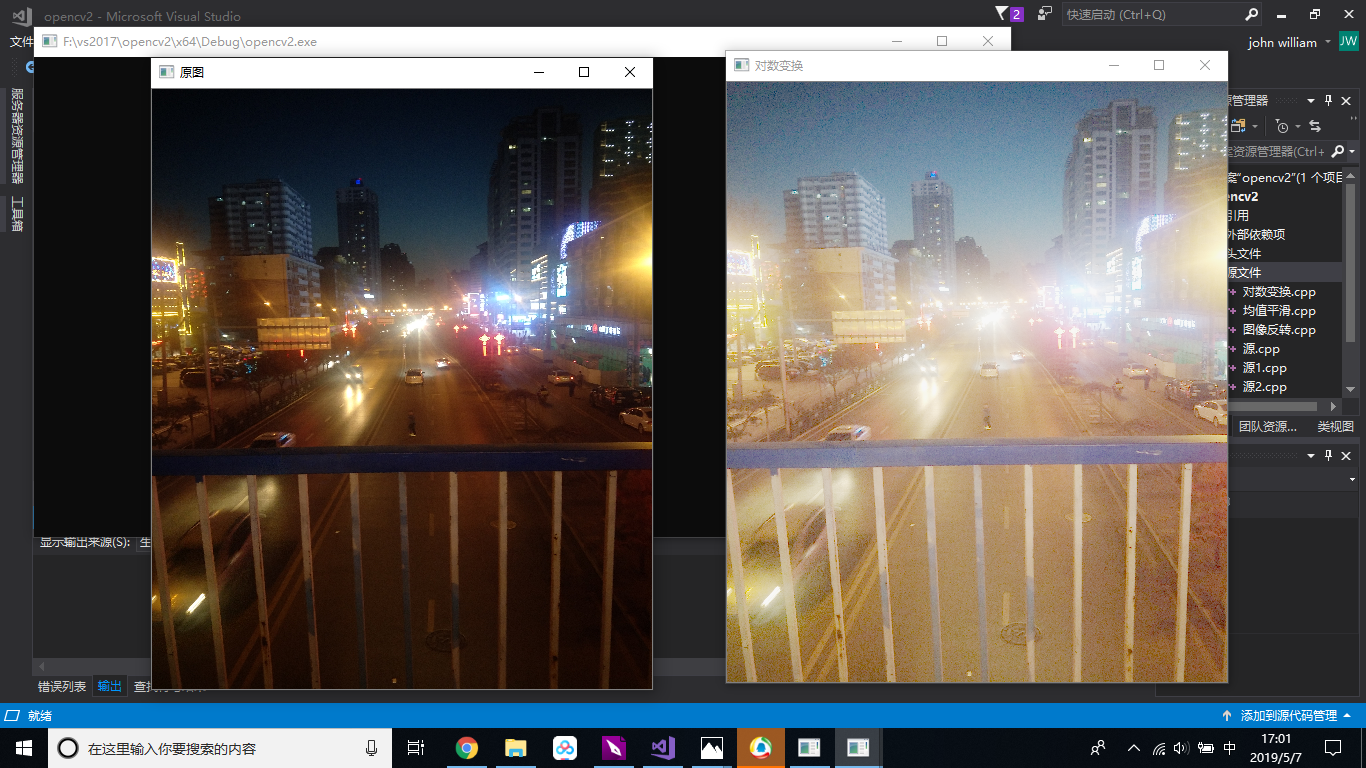展开全文• 对数变换： CImg&lt;int&gt; SrcImg; SrcImg.load_tiff("E:/Desktop/picture_process/Lenna/3/Fig0305(a)(DFT_no_log).tif"); SrcImg.display(); cimg_forXY(SrcImg, x, y) { SrcImg(x, y) = int...
对数变换：
CImg<int> SrcImg;
SrcImg.display();
cimg_forXY(SrcImg, x, y) {
SrcImg(x, y) = int(log(double(SrcImg(x, y)) + 1));
}
SrcImg.display();

运行效果：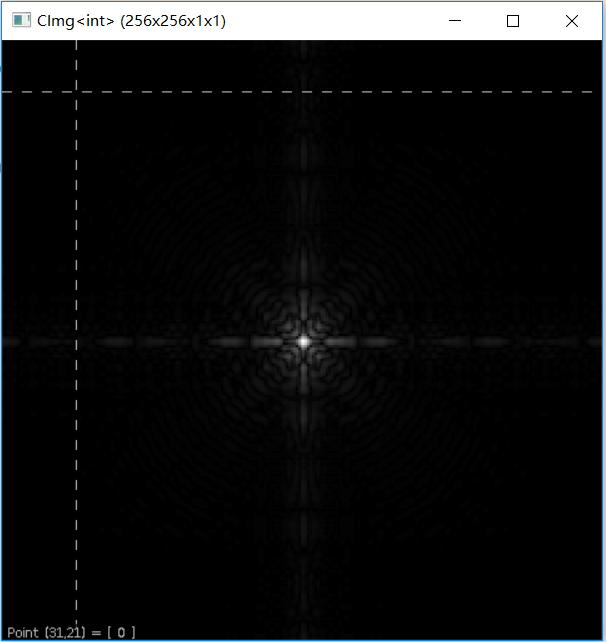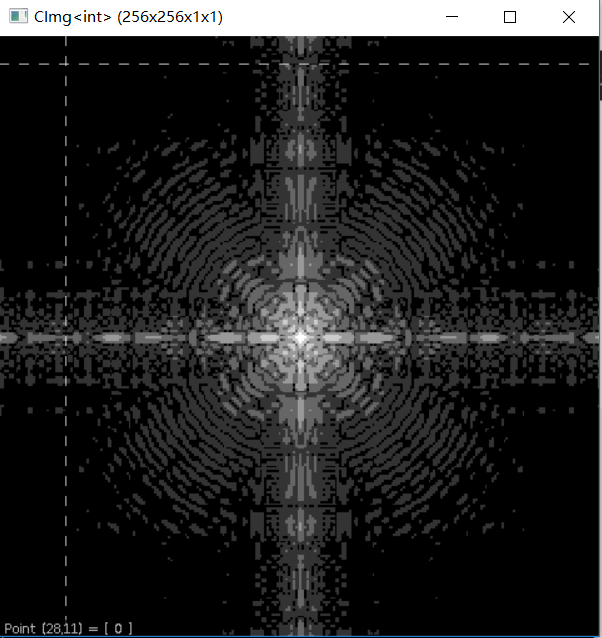幂律变换
CImg<int> SrcImg;
SrcImg.display();
double t = { 0.6,0.4,0.3 };
for (int i = 0; i < 3; i++) {
CImg<int> img = SrcImg;
cimg_forXY(img, x, y) {
img(x, y) = int(pow(double((img(x, y))), t[i]));
}
img.display();
}

运行结果：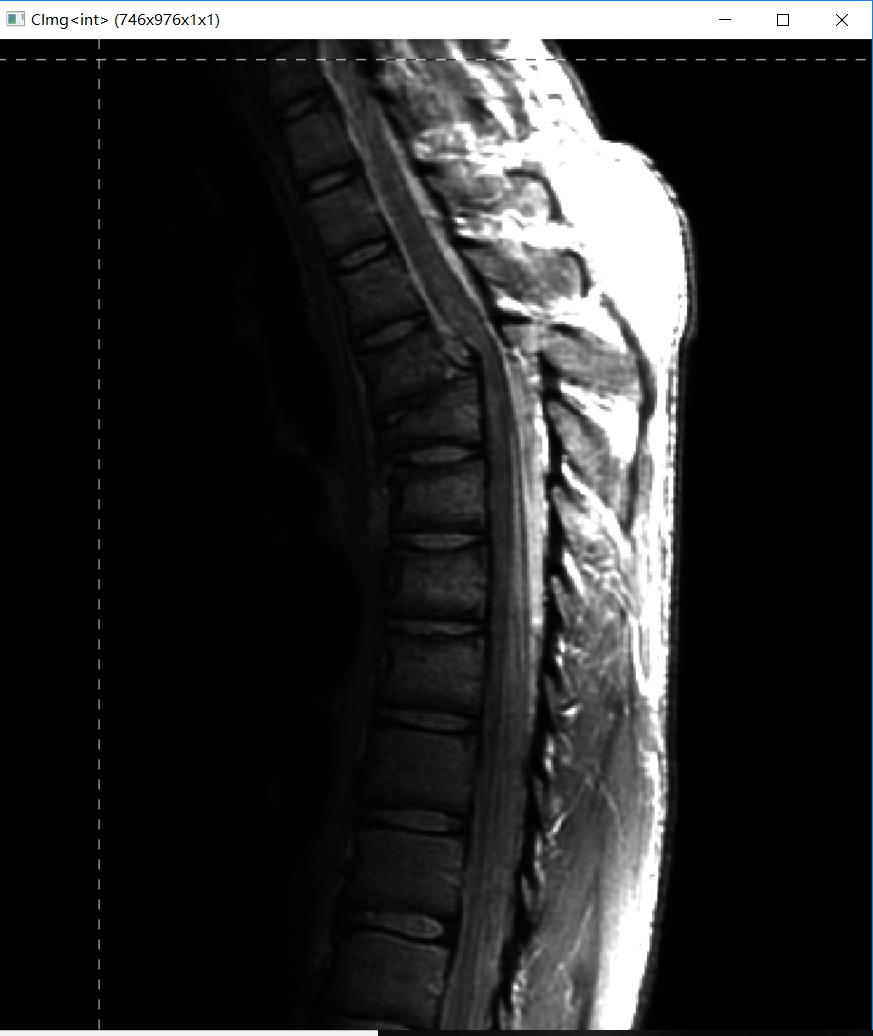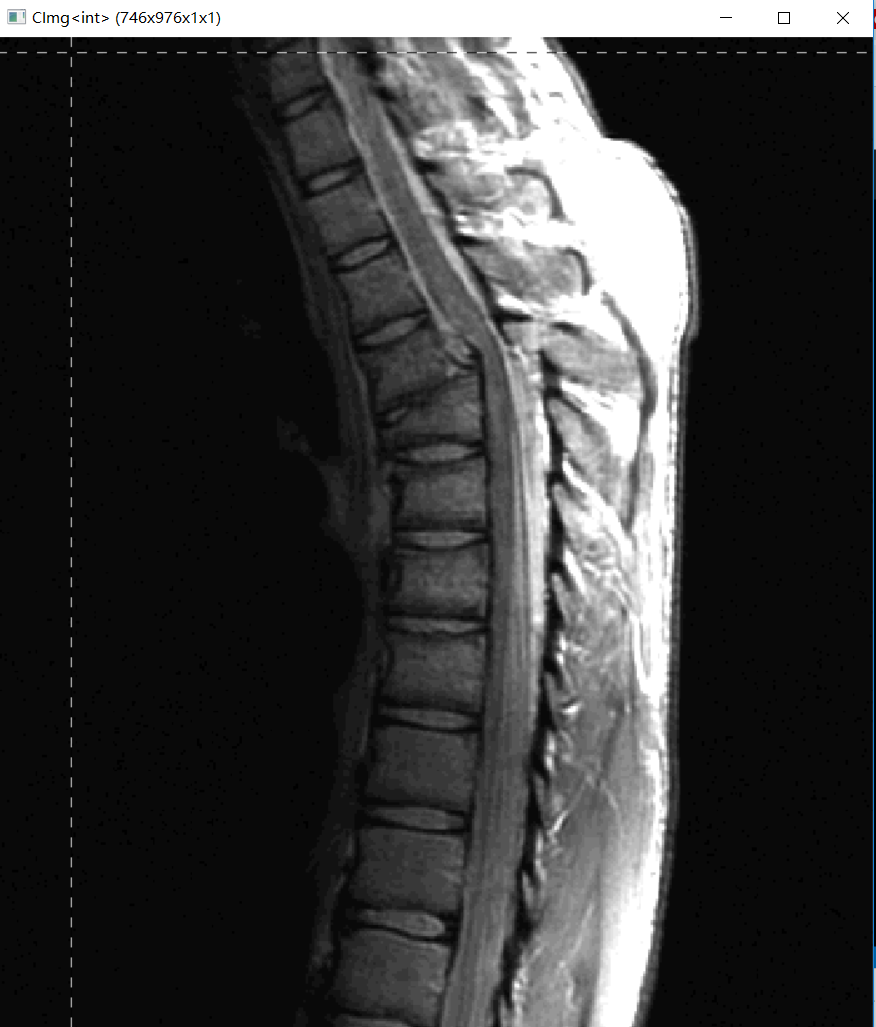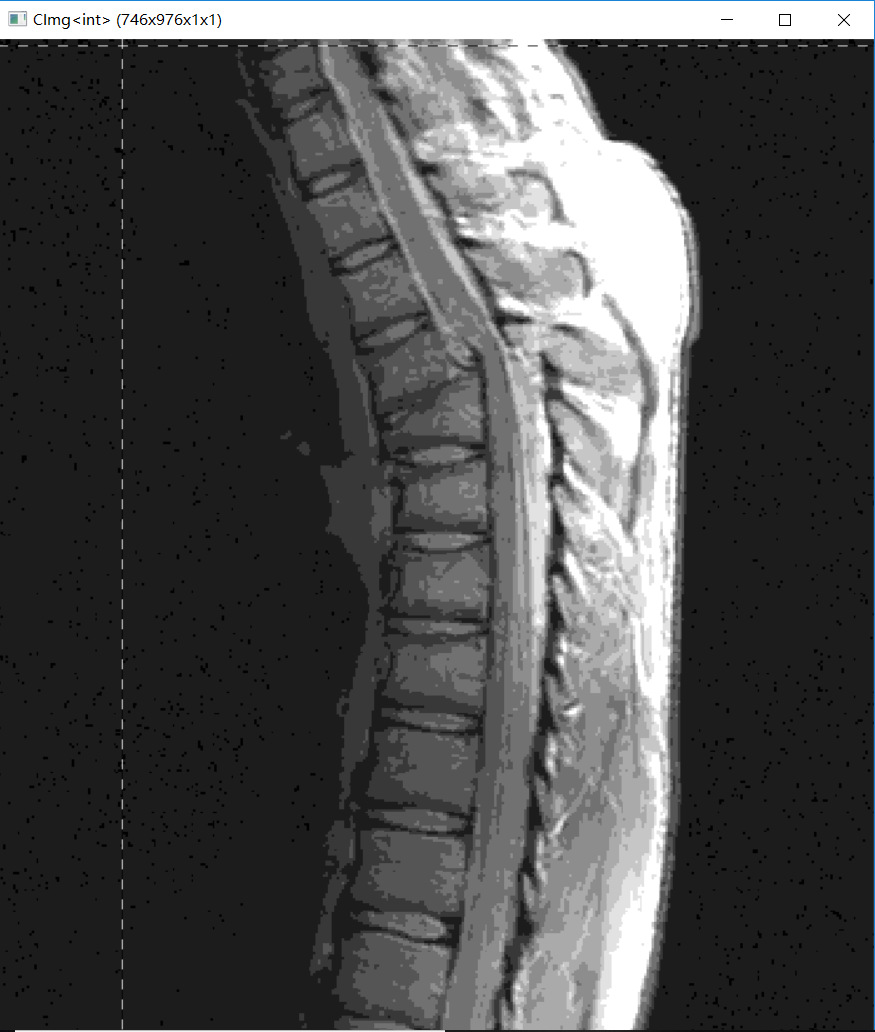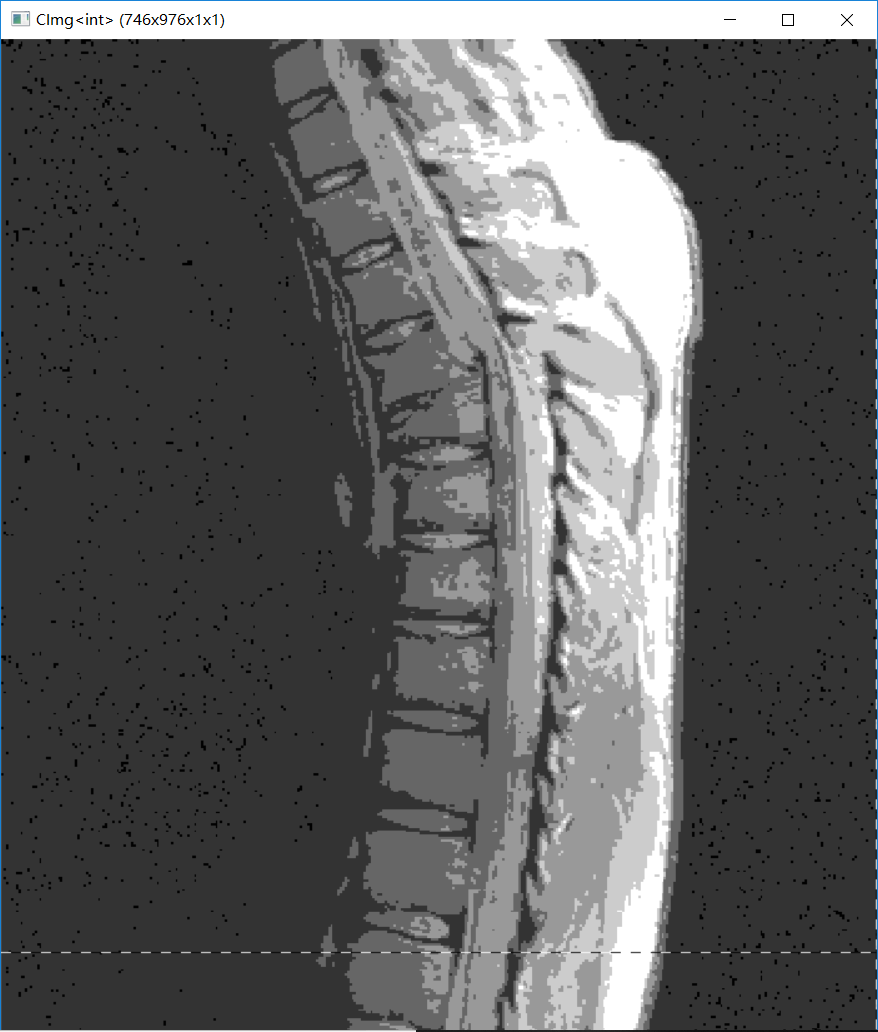比特面分层
CImg<int> img;
img.display("原图");
int w = img.height();
int h = img.width();
CImg<int> p1(h,w,1,1), p2(h, w, 1, 1), p3(h, w, 1, 1), p4(h, w, 1, 1), p5(h, w, 1, 1), p6(h, w, 1, 1), p7(h, w, 1, 1), p8(h, w, 1, 1);
cimg_forXY(img, x, y) {
int num = img(x, y);
int *bit = binary(num);
p1(x, y) = fx(bit); p2(x, y) = fx(bit); p3(x, y) = fx(bit); p4(x, y) = fx(bit);
p5(x, y) = fx(bit); p6(x, y) = fx(bit); p7(x, y) = fx(bit); p8(x, y) = fx(bit);
}
p8.display("第八层比特图");
p7.display("第七层比特图");
p6.display("第六层比特图");
p5.display("第五层比特图");
p4.display("第四层比特图");
p3.display("第三层比特图");
p2.display("第二层比特图");
p1.display("第一层比特图");
}
//用于对图片进行黑白的赋值
int fx(int n) {
if (n == 1)
return 255;
else
return 0;
}
//用于进行二进制转化的函数
int* binary(int num) {
int *bit;
bit = new int;
for (int i = 0; i < 8; i++) {
bit[i] = 0;
}
int i = 0;
while (num) {
bit[i] = num % 2;
num /= 2;
i++;
}
return bit;
}

运行结果：在这里插入图片描述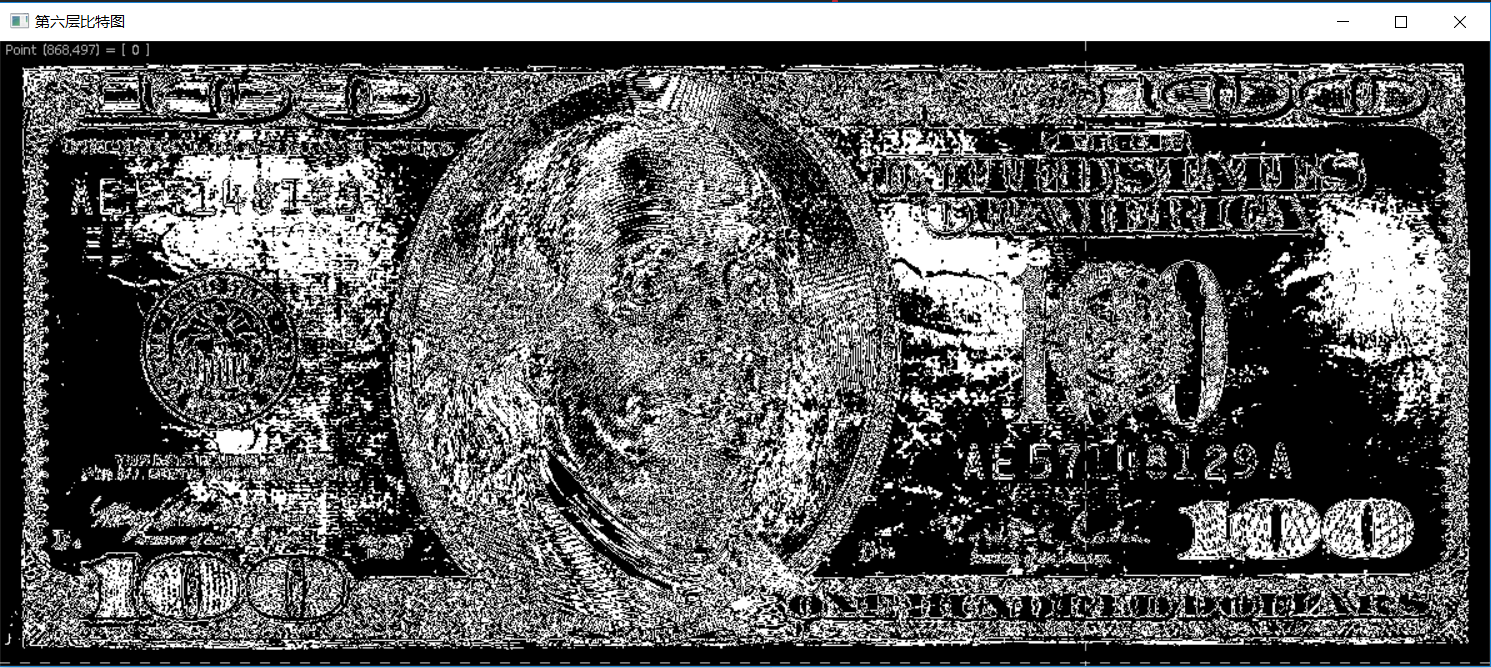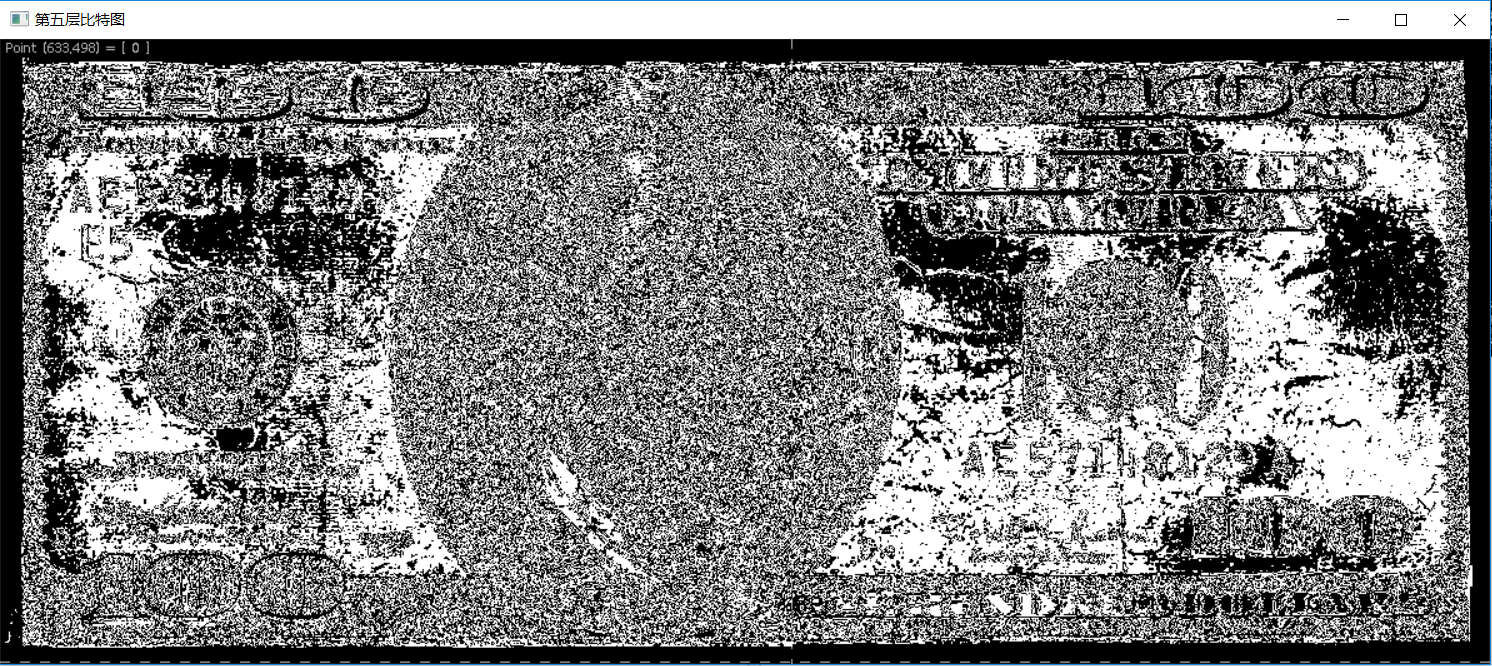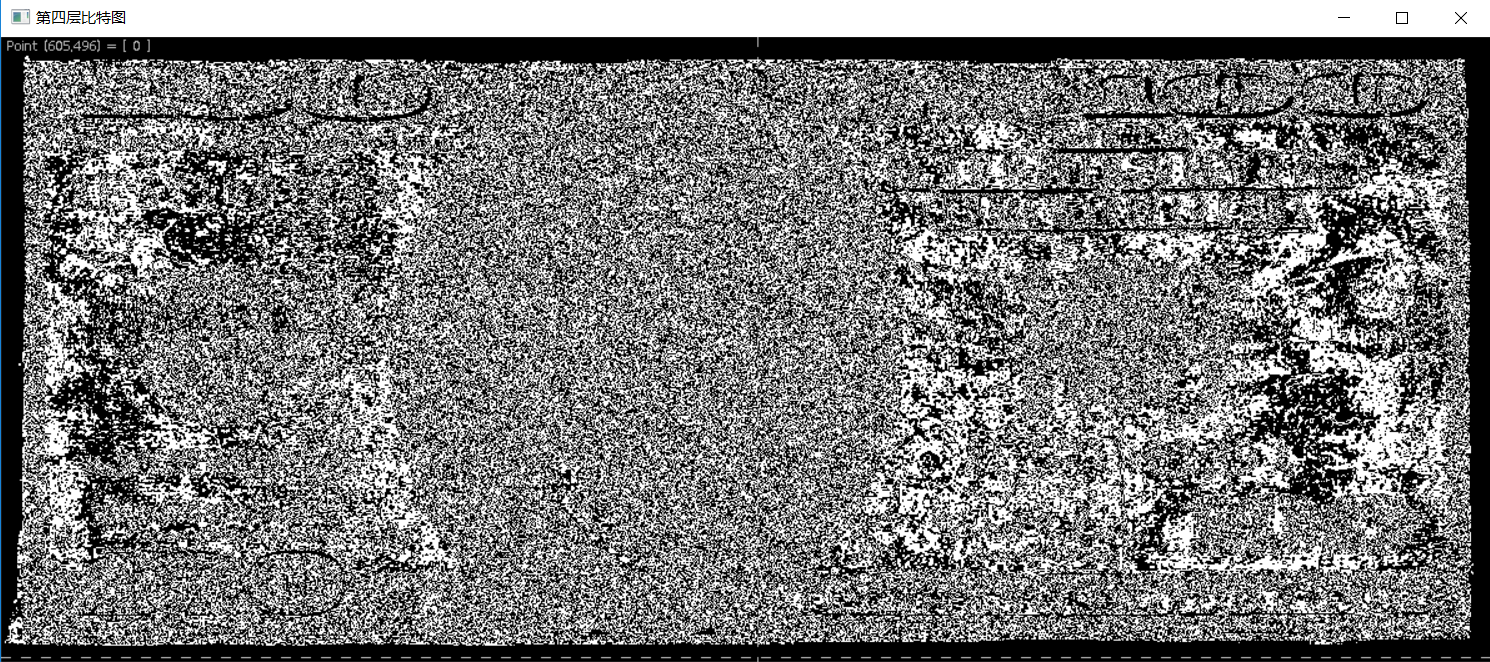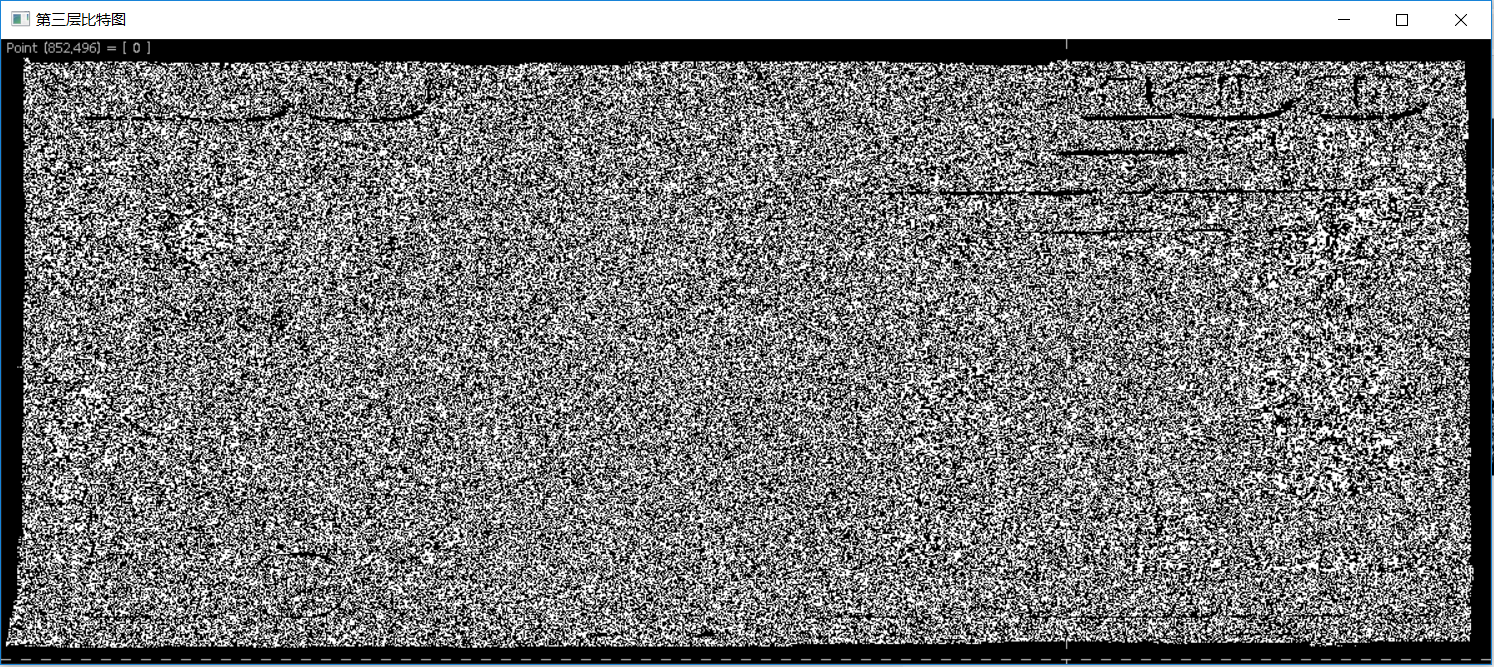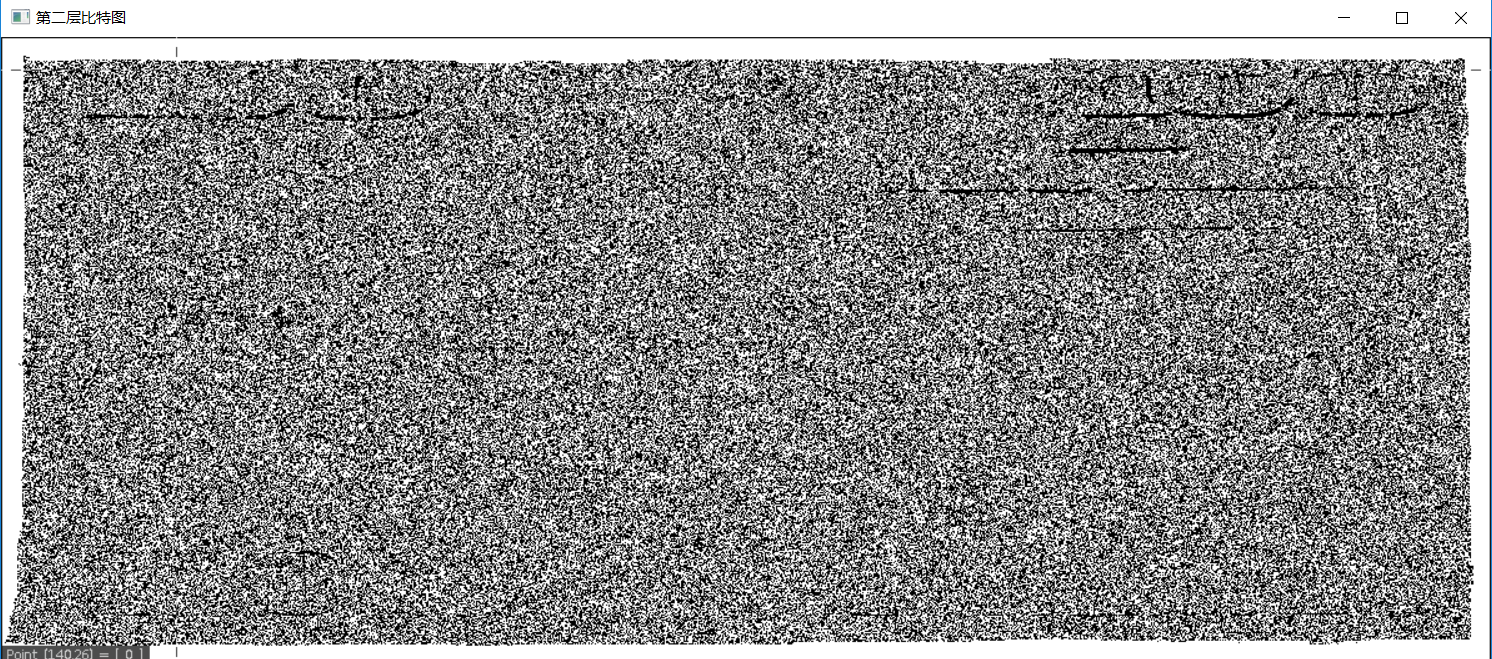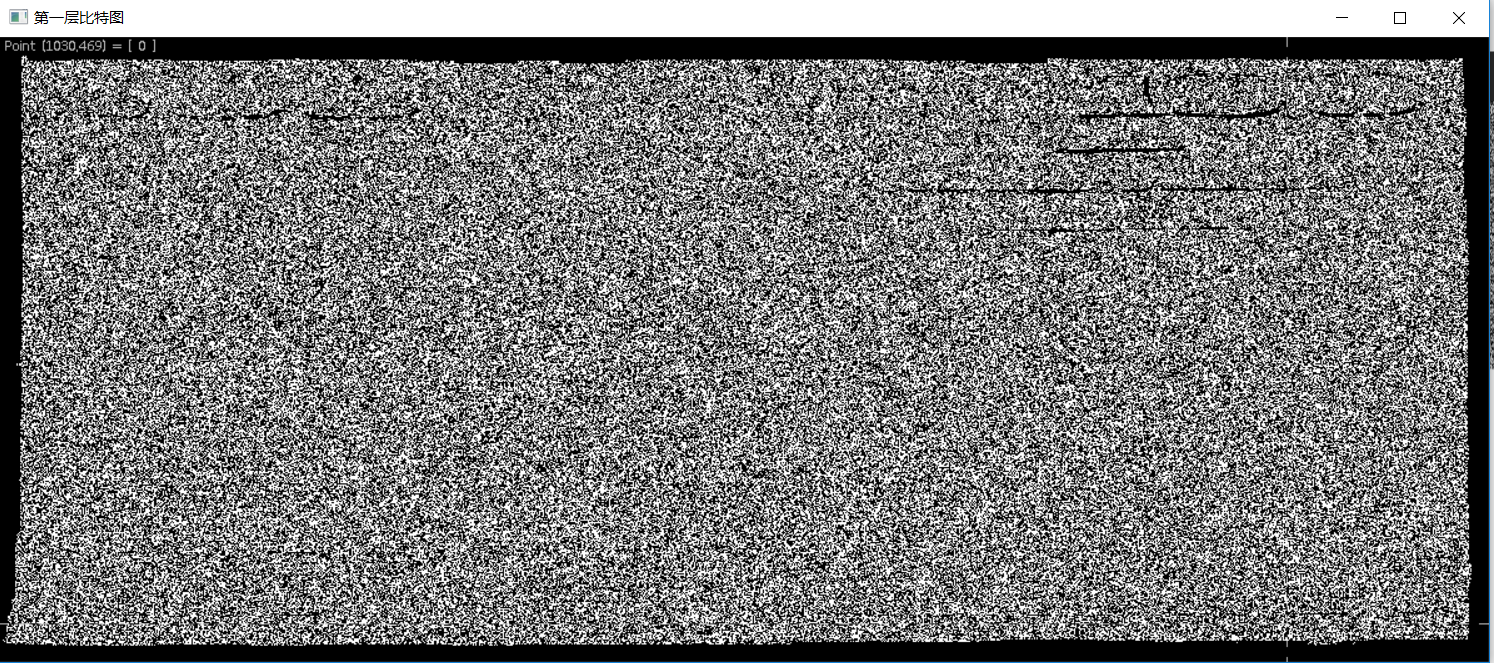均衡化处理

CImg有专门显示密度分布直方图的histogram函数

	CImg<int> hist = img.histogram(256, 0, 255); //histogram的第一个参数是共有256个灰度级，第二个参数是灰度的最小值，第三个是灰度最大值
hist.display_graph();

	CImg<int> img;
CImg<int> pic = img;
img.display("处理前图片");
int size = img.size();
CImg<int> hist = img.histogram(256, 0, 255); //histogram的第一个参数是共有256个灰度级，第二个参数是灰度的最小值，第三个是灰度最大值
hist.display_graph("处理前直方图");
double sum = 0.0;
map<int, int> index;
for (int i = 0; i < 256; i++) {
sum += (double) hist(i)/size;
int Sum = (int)(sum * 255);
index.insert(pair<int, int>(i, Sum));
}
//map<int, int>::iterator iter = index.begin();
//map<int, int>::iterator end = index.end();
/*for (; iter != end; iter++) {
cout << iter->first<<":"<< iter->second << endl;
}*/
cimg_forXY(pic, x, y) {
pic(x, y) = index[pic(x, y)];
//cout << pic(x, y) << " ";
}
pic.display("处理后图片");
CImg<int> hist1 = pic.histogram(256, 0, 255);
hist1.display_graph("处理后直方图");

运行结果：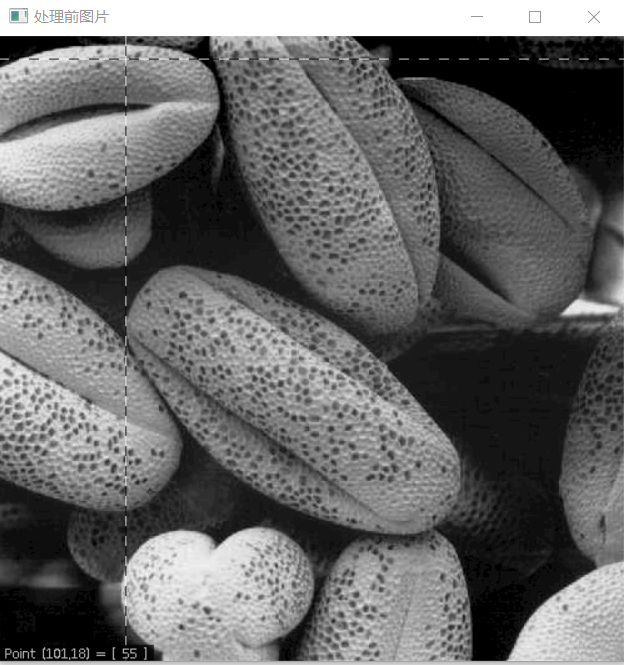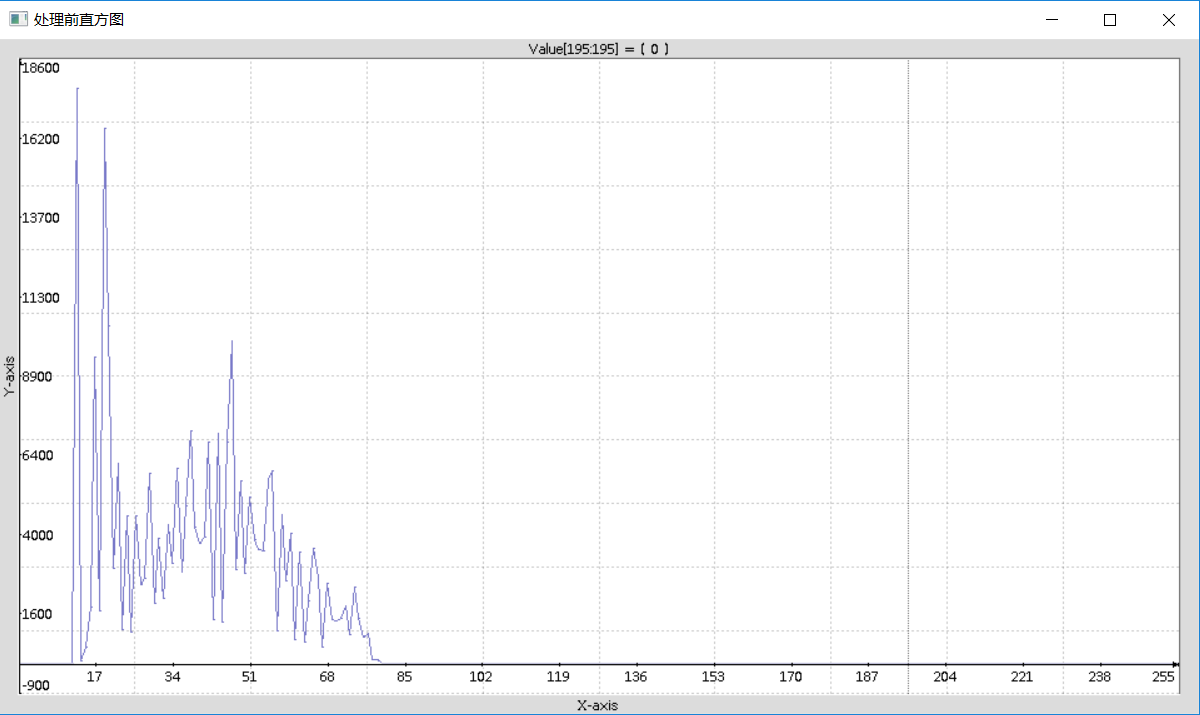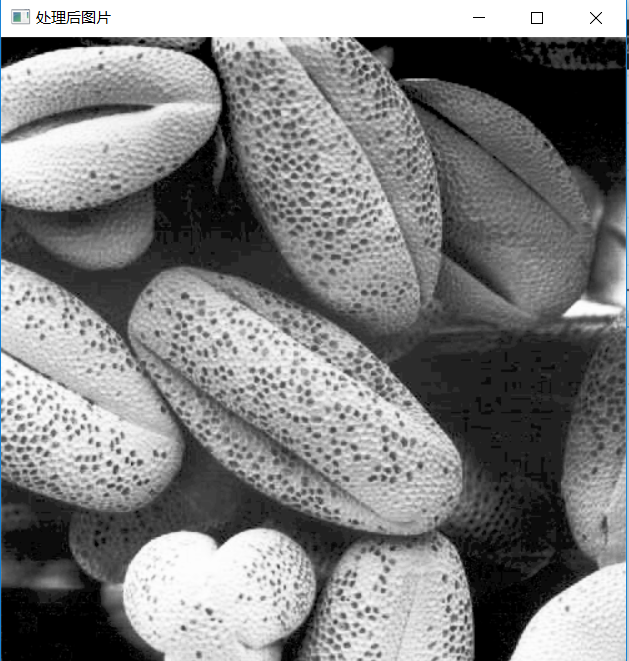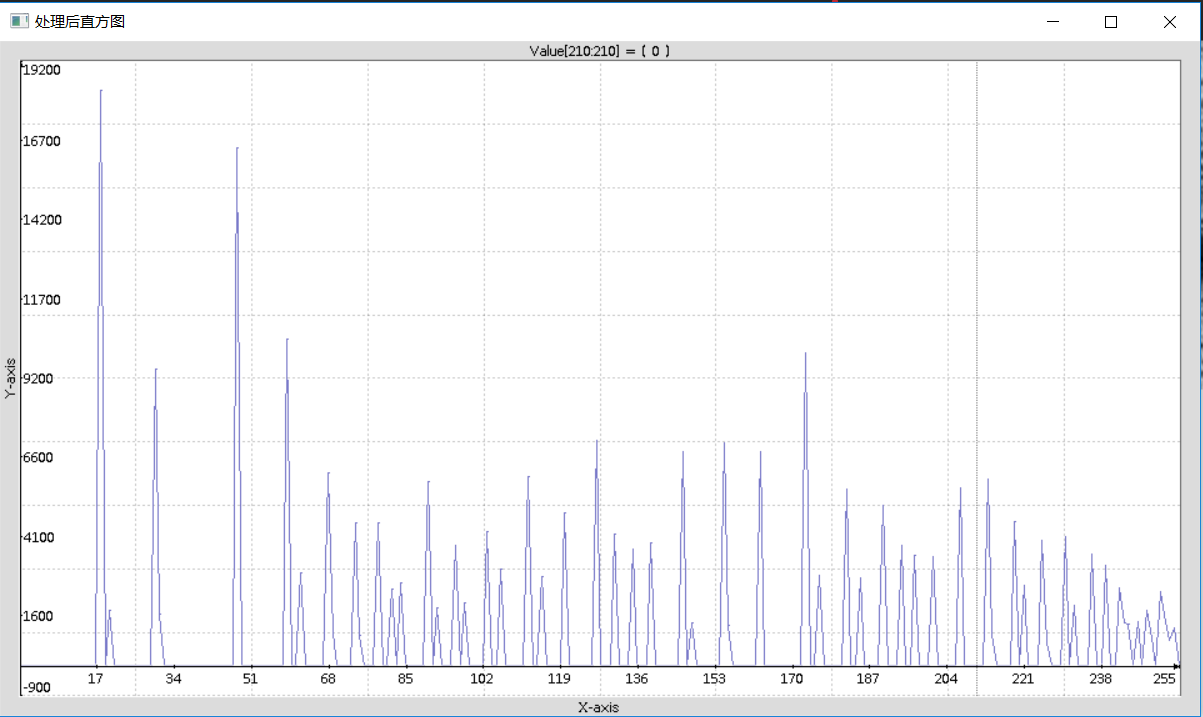展开全文CImg c++
• 对数变换可以拉伸范围较窄的低灰度值，同时压缩范围较宽的高灰度值。可以用来扩展图像中的暗像素值，同时压缩亮像素值。简而言之是对图像中低灰度细节进行增强其中 为常数， , 可以使函数左移一个单位，得到的s均...
对数变换可以拉伸范围较窄的低灰度值，同时压缩范围较宽的高灰度值。可以用来扩展图像中的暗像素值，同时压缩亮像素值。简而言之是对图像中低灰度细节进行增强其中为常数，,可以使函数左移一个单位，得到的s均大于0。'''对数变换'''import numpy as npimport matplotlib.pyplot as pltimport cv2def log_plot(c):x = np.arange(0, 256, 0.01)y = c*np.log(1 + x)plt.plot(x, y, 'r', linewidth=1)plt.title('对数变换函数')plt.xlim(0, 255), plt.ylim(0, 255)plt.show()def log(c, img):output_img = c*np.log(1.0+img)output_img = np.uint8(output_img+0.5)return output_imgimg_input = cv2.imread('FilePath')cv2.imshow('input', img_input)log_plot(42)img_output = log(42, img_input)cv2.imshow('output', img_output)cv2.waitKey(0)cv2.destroyAllWindows()Gamma校正该函数多用在图像整体偏暗，扩展灰度级。另外一种情况是，图像有“冲淡”的外观(很亮白)需要压缩中高以下的大部分的灰度级。简而言之是整体灰度值进行改变。其中为符号为正的常数。作为常数通常情况取1，输入输出情况下范围一般为.如果，光照强度增强，称为gamma compression。如果，光照强度减弱，称为gamma expansion。'''Gamma校正'''import numpy as npimport matplotlib.pyplot as pltimport cv2def gamma_plot(c, v):x = np.arange(0, 256, 0.01)y = c*x**vplt.plot(x, y, 'r', linewidth=1)plt.title('伽马变换函数')plt.xlim([0, 255]), plt.ylim([0, 255])plt.show()def gamma(img, c, v):lut = np.zeros(256, dtype=np.float32)for i in range(256):lut[i] = c * i ** voutput_img = cv2.LUT(img, lut)output_img = np.uint8(output_img+0.5)return output_imgimg_input = cv2.imread('FilePath', cv2.IMREAD_GRAYSCALE)cv2.imshow('imput', img_input)gamma_plot(0.00000005, 4.0)img_output = gamma(img_input, 0.00000005, 4.0)cv2.imshow('output', img_output)cv2.waitKey(0)cv2.destroyAllWindows()附上py3代码及matlab代码% i0为图像数据：% i1 = log(i+1);%对数变换，只能处理double类型值；% i1 = imadjust(i,[lin，hin],[lout，hout],r); gamma变换，[] 中可以定义范围，范围外的被裁剪掉，可缺省，r为gamma值；% i1 = im2bw(i,level); 阈值变换，level为阈值0-1；% thresh = graythresh(i); 自动确定阈值0-1；% i1 = im2bw(i,thresh); 利用上面的阈值处理，其中tresh为阈值取[0,1]i0 = imread('FilePath');i0 = im2double(i0);i1 = log(i0+1);i2 = imadjust(i,[],[],3);i3 = im2bw(i0,0.3);thresh = graythresh(i);i4 = im2bw(i,thresh);subplot(1,5,1); imshow(i0);subplot(1,5,2); imshow(i);subplot(1,5,3); imshow(i2);subplot(1,5,4); imshow(i3);subplot(1,5,5); imshow(i4);
展开全文• 基于FPGA的灰度图像处理对数变换 1 背景知识 对数变化的通用公式：s = clog(1+r)--------------------------------------------------------------(1) 其中c是一个常数，r为灰度级。 图1 一些基本的灰度变换函数...log
• 对数变换将输入中范围较窄的低灰度值映射为输出中范围较宽的灰度值，或将输入...使用对数变换来扩展图像中的暗像素值，同时压缩更高灰度级的值。反对数函数的作用于此相反。 对数变换的通用形式为： 对书中傅里叶频...
• 3.1 对数变换 3.2 伽马变换 参考资料 1 灰度变换简介 灰度变换是图像增强的一种重要手段，用于改善图像显示效果，属于空间域处理方法，它可以使图像动态范围加大，使图像对比度扩展，图像更加清晰，特征更加明显...Python OpenCV
• 作者 | 杨秀璋来源 | CSDN博客责编 | 夕颜头图 | 付费下载自视觉中国出品 | CSDN(ID:CSDNnews)本篇文章主要讲解非线性变换，使用自定义方法对图像进行灰度化处理，包括对数变换和伽马变换。本文主要讲解灰度线性变换...
• 本文参考了 以下这篇文章[数字图像处理]灰度变换——反转，对数变换，伽马变换，灰度拉伸，灰度切割，位图切割 https://blog.csdn.net/zhoufan900428/article/details/12709361并进行了一下改进。以上为上述文章 的...
• 数字图像处理-空间与处理-灰度变换-基本灰度变换函数（求反、线性灰度变换、换、对数变换、伽马变换、阈值化/二值化） [Python图像处理] 十五.图像的灰度线性变换 图像增强：线性、 分段线性、 对数、 反对数、 幂律...python opencv
• 作者 | 杨秀璋来源 | CSDN博客责编 | 夕颜头图 | 付费下载自视觉中国出品 | CSDN(ID:CSDNnews)本篇文章主要讲解非线性变换，使用自定义方法对图像进行灰度化处理，包括对数变换和伽马变换。本文主要讲解灰度线性变换...
• 目录一些基本的灰度变换函数图像反转对数变换 一些基本的灰度变换函数 如下图显示了在图像处理中频繁使用的3类基本函数： 线性（反转和恒等变换）函数 对数（对数和反对数变换）函数 幂律（nnn次幂和nnn次根变换）...
• [图像处理]_对数变换 算法实现 对数变换可实现图像的水平平移、竖直平移、对称变换等操作 也可实现图像灰度的扩展和压缩功能。 实现代码如下：  int LogTranslation(Mat srcImg, Mat dstImg, float a, float b, ...
• 数字图像处理-空间域处理-灰度变换-基本灰度变换函数（反转变换、对数变换、伽马变换和分段线性变换）
• ## Matlab 图像增强（对数变换）

万次阅读 多人点赞 2019-03-21 11:30:46
图像的对数变换： 通过灰度变换函数，调整输入低质图像的灰度值范围。将图像的低灰度值部分扩展，高灰度值部分压缩。...下面先介绍冈萨雷斯《数字图像处理》中设计的方法，我认为对图像的灰度提高的过于明...Matlab log
• 作者 | 杨秀璋来源 | CSDN博客责编 | 夕颜头图 | 付费下载自视觉中国出品 | CSDN(ID:CSDNnews)本篇文章主要讲解非线性变换，使用自定义方法对图像进行灰度化处理，包括对数变换和伽马变换。本文主要讲解灰度线性变换...
• 图像的灰度非线性变换之对数变换、伽马变换发布时间：2019-03-31 15:31,浏览次数：2077, 标签：Python该系列文章是讲解PythonOpenCV图像处理知识，前期主要讲解图像入门、OpenCV基础用法，中期讲解图像处理的各种...
• 空间域增强的第一部分：图像反转，对数变换，幂次变换、分段线性变换图像增强
• 作者 | 杨秀璋来源 | CSDN博客责编 | 夕颜头图 | 付费下载自视觉中国出品 | CSDN(ID:CSDNnews)本篇文章主要讲解非线性变换，使用自定义方法对图像进行灰度化处理，包括对数变换和伽马变换。本文主要讲解灰度线性变换...
• 1.图像的线性变换 图像的线性变换 S=k×r+b S=k\times r+b S=k×r+b 其中 rrr 为输入变量，SSS为输出变量，k，bk，bk，b为线性变换中的倍数因子和偏移因子。 当0<k<10<k<10<k<1时，对灰度压缩，...opencv python...